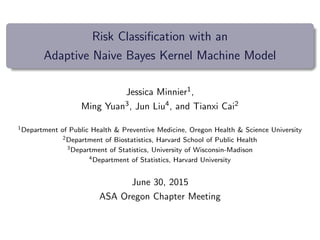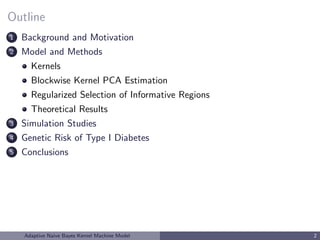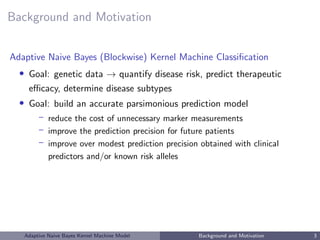Successfully reported this slideshow.

# Risk Classification with an Adaptive Naive Bayes Kernel Machine Model

2

Share×
1 of 36
1 of 36

# Risk Classification with an Adaptive Naive Bayes Kernel Machine Model

2

Share

ASA Oregon Chapter Meeting Talk

ASA Oregon Chapter Meeting Talk

## More Related Content

### Related Books

Free with a 30 day trial from Scribd

See all

### Related Audiobooks

Free with a 30 day trial from Scribd

See all

### Risk Classification with an Adaptive Naive Bayes Kernel Machine Model

1. 1. Risk Classiﬁcation with an Adaptive Naive Bayes Kernel Machine Model Jessica Minnier1, Ming Yuan3, Jun Liu4, and Tianxi Cai2 1Department of Public Health & Preventive Medicine, Oregon Health & Science University 2Department of Biostatistics, Harvard School of Public Health 3Department of Statistics, University of Wisconsin-Madison 4Department of Statistics, Harvard University June 30, 2015 ASA Oregon Chapter Meeting
2. 2. Outline 1 Background and Motivation 2 Model and Methods Kernels Blockwise Kernel PCA Estimation Regularized Selection of Informative Regions Theoretical Results 3 Simulation Studies 4 Genetic Risk of Type I Diabetes 5 Conclusions Adaptive Naive Bayes Kernel Machine Model 2
3. 3. Background and Motivation Adaptive Naive Bayes (Blockwise) Kernel Machine Classiﬁcation • Goal: genetic data → quantify disease risk, predict therapeutic eﬃcacy, determine disease subtypes • Goal: build an accurate parsimonious prediction model – reduce the cost of unnecessary marker measurements – improve the prediction precision for future patients – improve over modest prediction precision obtained with clinical predictors and/or known risk alleles Adaptive Naive Bayes Kernel Machine Model Background and Motivation 3
4. 4. Background and Motivation Adaptive Naive Bayes (Blockwise) Kernel Machine Classiﬁcation • Goal: genetic data → quantify disease risk, predict therapeutic eﬃcacy, determine disease subtypes • Goal: build an accurate parsimonious prediction model – reduce the cost of unnecessary marker measurements – improve the prediction precision for future patients – improve over modest prediction precision obtained with clinical predictors and/or known risk alleles • Complex diseases – many alleles contribute to risk – many distinct combinations of risk factors lead to disease Adaptive Naive Bayes Kernel Machine Model Background and Motivation 3
5. 5. Background and Motivation • Genome wide association studies (GWAS) – identifying SNPs associated with disease risk – primary goal of testing – accurate risk prediction remains diﬃcult • Common approach: – select top ranked SNPs based on large scale testing – construct a composite genetic score w/ selected SNPs Adaptive Naive Bayes Kernel Machine Model Background and Motivation 4
6. 6. Background and Motivation • Genome wide association studies (GWAS) – identifying SNPs associated with disease risk – primary goal of testing – accurate risk prediction remains diﬃcult • Common approach: – select top ranked SNPs based on large scale testing – construct a composite genetic score w/ selected SNPs – may not work well due to false +/− errors in identifying predictive SNPs over-ﬁtting using only subset of SNPs available additive eﬀects only Adaptive Naive Bayes Kernel Machine Model Background and Motivation 4
7. 7. Background and Motivation Recent progress in prediction with high dimensional data • Regularized estimation: LASSO (Tibshirani, 1996); SCAD (Fan and Li, 2001); Adaptive LASSO (Zou, 2006) • Machine learning: Support vector machine (Cristianini, Shawe-Taylor, 2000); Least square Kernel Machine Regression (Liu, Lin, Ghosh, 2007); Kernel logistic regression (Zhu and Hastie, 2005; Liu, Ghosh and Lin, 2008) • Screening + Regularized estimation: Sure independence screening (Fan and Lv, 2008; Fan and Song, 2009) Global methods: may be unstable for large p, high correlation Adaptive Naive Bayes Kernel Machine Model Background and Motivation 5
8. 8. Approach Challenge: • Prediction models based on univariate testing, additive models, global methods → low prediction accuracy, low AUC, missing heritability • Non-linear eﬀects? testing for interactions → low power Adaptive Naive Bayes Kernel Machine Model Model and Methods 6
9. 9. Approach Challenge: • Prediction models based on univariate testing, additive models, global methods → low prediction accuracy, low AUC, missing heritability • Non-linear eﬀects? testing for interactions → low power Our approach [Minnier et al., 2015]: • Blockwise method: leverage biological knowledge to build models at the gene-set level genes, gene-pathways, linkage disequilibrium blocks • Kernel machine regression: allow for complex and nonlinear eﬀects implicitly specify underlying complex functional form of covariate eﬀects via similarity measures (kernels) that deﬁne the distance between two sets of covariates Adaptive Naive Bayes Kernel Machine Model Model and Methods 6
10. 10. Kernel Methods: similar inputs to similar outputs • transform data to feature space H with non-linear map φ • “kernel trick” lets us use K(, ) similarity function instead of φ • K induces the feature space N. Takahashi’s webpage Adaptive Naive Bayes Kernel Machine Model Model and Methods 7
11. 11. Previous Methods Blockwise methods • Inference: Gene-set testing Gene burden tests Gene Set Enrichment Analysis (GSEA) SNP-set Sequence Kernel Association Test (SKAT, SKAT-O; Wu et al. 2010; Wu, Lee, et al. 2011) Adaptive Naive Bayes Kernel Machine Model Model and Methods 8
12. 12. Previous Methods Blockwise methods • Inference: Gene-set testing Gene burden tests Gene Set Enrichment Analysis (GSEA) SNP-set Sequence Kernel Association Test (SKAT, SKAT-O; Wu et al. 2010; Wu, Lee, et al. 2011) Kernel machine methods • Support Vector Machine (SVM) classiﬁcation methods • Inference KM SNP-set Testing (Liu et al. 2007, 2008; SKAT methods) Gene expression test with kernel Cox model (Li and Luan 2003) Adaptive Naive Bayes Kernel Machine Model Model and Methods 8
13. 13. Notations and Model Assumptions • Data – Response: Y = (Y1, ..., Yn)T – Predictors: M blocks of genomic regions, for b = 1, ..., M, X(b) = (X (b) 1 , ..., X(b) n )T n×pb , Adaptive Naive Bayes Kernel Machine Model Model and Methods 9
14. 14. Notations and Model Assumptions • Data – Response: Y = (Y1, ..., Yn)T – Predictors: M blocks of genomic regions, for b = 1, ..., M, X(b) = (X (b) 1 , ..., X(b) n )T n×pb , • Blockwise: Partition genome into gene-sets – Recombination hotspots, gene-pathways Adaptive Naive Bayes Kernel Machine Model Model and Methods 9
15. 15. Notations and Model Assumptions • Data – Response: Y = (Y1, ..., Yn)T – Predictors: M blocks of genomic regions, for b = 1, ..., M, X(b) = (X (b) 1 , ..., X(b) n )T n×pb , • Model under blockwise Naive Bayes (NB) assumption: X(1) , ..., X(M) | Y independent Adaptive Naive Bayes Kernel Machine Model Model and Methods 10
16. 16. Notations and Model Assumptions • Data – Response: Y = (Y1, ..., Yn)T – Predictors: M blocks of genomic regions, for b = 1, ..., M, X(b) = (X (b) 1 , ..., X(b) n )T n×pb , • Model under blockwise Naive Bayes (NB) assumption: X(1) , ..., X(M) | Y independent ⇒ logit{pr(Y = 1 | X(1) , ..., X(M) )} = c + M b=1 logit{pr(Y = 1 | X(b) )} – NB assumption allows separate estimation by block and reduces overﬁtting – Performs well for zero-one loss L(X) = I( ˆY (X) = Y ) [Domingos and Pazzani, 1997] Adaptive Naive Bayes Kernel Machine Model Model and Methods 10
17. 17. Notations and Model Assumptions • Within each region, the eﬀect may be complex and interactive due to – multiple causal variants – un-typed causal variants in the presence of high LD Adaptive Naive Bayes Kernel Machine Model Model and Methods 11
18. 18. Notations and Model Assumptions • Within each region, the eﬀect may be complex and interactive due to – multiple causal variants – un-typed causal variants in the presence of high LD • Blockwise Kernel Machine Regression logit{pr(Y = 1 | X(b) )} = a(b) +h(b) (X(b) ) h(b) (X(b) ) = l β(b) l ψ(b) l (X(b) ) ∈ HK(b) {ψ(b) l } = { λ(b) l φ(b) l } implicitly speciﬁed via a symmetric positive deﬁnite kernel K(b) (·, ·). Adaptive Naive Bayes Kernel Machine Model Model and Methods 11
19. 19. Notations and Model Assumptions • Within each region, the eﬀect may be complex and interactive due to – multiple causal variants – un-typed causal variants in the presence of high LD • Blockwise Kernel Machine Regression logit{pr(Y = 1 | X(b) )} = a(b) +h(b) (X(b) ) h(b) (X(b) ) = l β(b) l ψ(b) l (X(b) ) ∈ HK(b) {ψ(b) l } = { λ(b) l φ(b) l } implicitly speciﬁed via a symmetric positive deﬁnite kernel K(b) (·, ·). K(b) (X(b) i , X(b) j ) deﬁnes the similarity between X(b) i and X(b) j . Adaptive Naive Bayes Kernel Machine Model Model and Methods 11
20. 20. Notations and Model Assumptions • Within each region, the eﬀect may be complex and interactive due to – multiple causal variants – un-typed causal variants in the presence of high LD • Blockwise Kernel Machine Regression logit{pr(Y = 1 | X(b) )} = a(b) +h(b) (X(b) ) h(b) (X(b) ) = l β(b) l ψ(b) l (X(b) ) ∈ HK(b) {ψ(b) l } = { λ(b) l φ(b) l } implicitly speciﬁed via a symmetric positive deﬁnite kernel K(b) (·, ·). K(b) (X(b) i , X(b) j ) deﬁnes the similarity between X(b) i and X(b) j . HK(b) , the functional space spanned by K(b) (·, ·), is a reproducible kernel hilbert space (RKHS) Adaptive Naive Bayes Kernel Machine Model Model and Methods 11
21. 21. Choices of Kernel Functions Linear kernel: K(Xi , Xj ) = ρ + XT i Xj , h(X) = p k=1 βkXk Fitting logistic regression with linear kernel ⇔ logistic ridge regression. Adaptive Naive Bayes Kernel Machine Model Model and Methods 12
22. 22. Choices of Kernel Functions Linear kernel: K(Xi , Xj ) = ρ + XT i Xj , h(X) = p k=1 βkXk Fitting logistic regression with linear kernel ⇔ logistic ridge regression. IBS kernel: K(Xi , Xj ) = p k=1(2 − |Xik − Xjk|), powerful in detecting non-linear eﬀects with SNP data [Wu et al, 2010] Adaptive Naive Bayes Kernel Machine Model Model and Methods 12
23. 23. Estimation of h: Kernel PCA • primal form: h = l βl ψl = l βl √ λl φl • Kernel PCA approximation: K = [K(Xi , Xj )]1≤i,j≤n = n l=1 λl φl φ T l K = 0 l=1 λl φl φ T l = ΨΨ T ; Ψ = [λ 1 2 1 φ1, ..., λ 1 2 0 φ 0 ]n× 0 Scholkopf et al. ; Williams and Seeger ; Braun et al. ; Zhang et al.  • h(b) (X(b) ) = Ψβ • obtain (a, β) as the minimizer of ridge logistic objective function L(Y , a, Ψβ) + τ β 2 Adaptive Naive Bayes Kernel Machine Model Model and Methods 13
24. 24. Regularized Selection of Informative Regions • For b = 1, ..., M, perform kernel PCA regression and obtain h(b) logit{pr(Y = 1 | X(b) )} = a(b) + h(b) (X(b) ) • Classify a future subject with X = {X(b) , b = 1, ..., M} based on M b=1 h(b) (X(b) ) ≥ c • Final prediction rule with weighted block eﬀects – Some regions may not be predictive of the outcome due to false discovery – Inclusion of all regions for prediction may lead to reduced accuracy – Regularized estimation of block eﬀects using LASSO: M b=1 γbh(b) (X(b) ) ≥ c Adaptive Naive Bayes Kernel Machine Model Model and Methods 14
25. 25. Regularized Selection of Informative Regions • For b = 1, ..., M, perform kernel PCA regression and obtain h(b) logit{pr(Y = 1 | X(b) )} = a(b) + h(b) (X(b) ) • Classify a future subject with X = {X(b) , b = 1, ..., M} based on M b=1 h(b) (X(b) ) ≥ c • Final prediction rule with weighted block eﬀects – Regularized estimation of block eﬀects using LASSO, pseudo-data H estimated with cross-validation: K k=1 YT log g(b + Hγ) + (1 − Y)T log{1 − g(b + Hγ)} − τ2 γ 1, M b=1 γbh(b) (X(b) ) ≥ c Adaptive Naive Bayes Kernel Machine Model Model and Methods 15
26. 26. Theoretical Results • Consistency of h(b)(x): – h(b) (x) → h(b) (x) at √ n rate for ﬁnite dimensional HK – Relies on convergence of sample eigen-values and -vectors from kernel PCA to the true eigensystem of HK Ψ → Ψ = {ψ(b) 1 , . . . , ψ(b) 0 } • Oracle property of γ: – Gene-set selection consistency P(A = A) → 1 where A = {b|h(b) (x) = 0}, A = {b|h(b) (x) = 0} Adaptive Naive Bayes Kernel Machine Model Model and Methods 16
27. 27. Simulation Studies for NBKM • SNP data sampled from gene-sets in a GWAS dataset (from type I diabetes study, Aﬀy 500k) • 350 regions, 9256 SNPs • Only the ﬁrst 4 regions are associated with the outcome • the joint eﬀects of the SNPs in each of these regions set as – linear for the ﬁrst two regions and non-linear for the other 2 regions – linear for all 4 regions – nonlinear for all 4 regions Adaptive Naive Bayes Kernel Machine Model Simulation Studies 17
28. 28. Prediction Accuracy Simulations: nt = 1000, nv = 500, # of genes = 350 total # of SNPs = 9256 Adaptive Naive Bayes Kernel Machine Model Simulation Studies 18
29. 29. Gene-set selection Simulations: nt = 1000, nv = 500, # of genes = 350 total # of SNPs = 9256 Adaptive Naive Bayes Kernel Machine Model Simulation Studies 19
30. 30. Genetic Risk of Type I Diabetes • Autoimmune disease, usually diagnosed in childhood • T1D – 75 SNPs have been identiﬁed as T1D risk alleles (National Human Genome Research Institute, Hindorﬀ et al. ) – 91 genes that either contain these SNPs or ﬂank the SNP on either side on the chromosome Adaptive Naive Bayes Kernel Machine Model Genetic Risk of Type I Diabetes 20
31. 31. Genetic Risk of Type I Diabetes • Autoimmune disease, usually diagnosed in childhood • T1D – 75 SNPs have been identiﬁed as T1D risk alleles (National Human Genome Research Institute, Hindorﬀ et al. ) – 91 genes that either contain these SNPs or ﬂank the SNP on either side on the chromosome • T1D + Other autoimmune diseases (Rheumatoid arthritis, Celiac disease, Crohns disease, Lupus, Inﬂammatory bowel disease) – 365 SNPs have been identiﬁed as T1D+other autoimmune disease risk alleles (NHGRI) – 375 genes that either contain these SNPs or ﬂank the SNP on either side on the chromosome Adaptive Naive Bayes Kernel Machine Model Genetic Risk of Type I Diabetes 20
32. 32. Genetic Risk of Type I Diabetes GWAS data collected by Welcome Trust Case Control Consortium (WTCCC) • 2000 cases, 3000 controls of European descent from Great Britain • segment the genome into gene-sets: gene and a ﬂanking region of 20KB on either side of the gene • The WTCCC data includes – 350 of the gene-sets listed in the NHGRI catalog – covering 9,256 SNPs in the WTCCC data Adaptive Naive Bayes Kernel Machine Model Genetic Risk of Type I Diabetes 21
33. 33. T1D Prediction Results Adaptive Naive Bayes Kernel Machine Model Genetic Risk of Type I Diabetes 22
34. 34. Conclusions • Kernel Machine Regression provides a useful tool for incorporating non-linear complex eﬀects • Blockwise KM regression achieves a nice balance between capturing complex eﬀects and overﬁtting • IBS kernel performs well under both linear and non-linear settings Remarks • May use SKAT to screen blocks for initial stage • Can be extended to data with other covariates such as clinical variables • Possible extensions might incorporate more complex block structure, diﬀerent types of outcomes, interactions, and beyond! Adaptive Naive Bayes Kernel Machine Model Conclusions 23
35. 35. Thank you! Adaptive Naive Bayes Kernel Machine Model Conclusions 24
36. 36. References I M. Braun, J. Buhmann, and K. M¨uller. On relevant dimensions in kernel feature spaces. The Journal of Machine Learning Research, 9:1875–1908, 2008. P. Domingos and M. Pazzani. On the optimality of the simple bayesian classiﬁer under zero-one loss. Machine learning, 29(2): 103–130, 1997. L. Hindorﬀ, P. Sethupathy, H. Junkins, E. Ramos, J. Mehta, F. Collins, and T. Manolio. Potential etiologic and functional implications of genome-wide association loci for human diseases and traits. Proceedings of the National Academy of Sciences, 106(23):9362, 2009. J. Minnier, M. Yuan, J. S. Liu, and T. Cai. Risk classiﬁcation with an adaptive naive bayes kernel machine model. Journal of the American Statistical Association, 110(509):393–404, 2015. B. Scholkopf, S. Mika, C. Burges, P. Knirsch, K. Muller, G. Ratsch, and A. Smola. Input space versus feature space in kernel-based methods. Neural Networks, IEEE Transactions on, 10(5):1000–1017, 1999. C. Williams and M. Seeger. The eﬀect of the input density distribution on kernel-based classiﬁers. In Proceedings of the 17th International Conference on Machine Learning. Citeseer, 2000. R. Zhang, W. Wang, and Y. Ma. Approximations of the standard principal components analysis and kernel pca. Expert Systems with Applications, 37(9):6531–6537, 2010. Adaptive Naive Bayes Kernel Machine Model 25